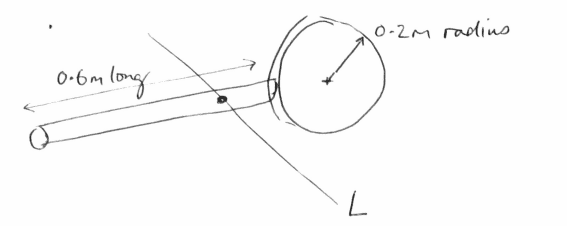# Determine the moment of inertia of a bar and disk assembly

TheBigDig
Homework Statement:
A homogeneous 3kg rod is welded to a homogeneous 2kg disc. Determine it's moment of inertia about the axis L which passes through the object's centre of mass. L is perpendicular to the bar and the disk.
Relevant Equations:
## I_x = \int y^2\mathrm{d}A ##
## I_y = \int x^2\mathrm{d}A ##I have been given an answer for this but I am struggling to get to that point
$$ANS = 0.430\, kg \cdot m^2$$

So I thought using the moment of inertia of a compound pendulum might work where ##I_{rod} = \frac{ml^2}{12}## and ##I_{disc} = \frac{mR^2}{2}## (##l## is the length of the rod and ##R## is the radius of the disc)
$$I_P = I_{rod} + M_{rod} \bigg( \frac{S}{2} \bigg)^2 + I_{disc} + M_{disc} (S+R)^2$$
where S is the length of the pendulum

$$I_P = 0.09 + 1.2 + 0.04 + 2 = 2.61\, kg \cdot m^2$$
Much too large for my purposes.
Not really sure where to go after this. Any help is appreciated.

Last edited:

Homework Helper
Gold Member